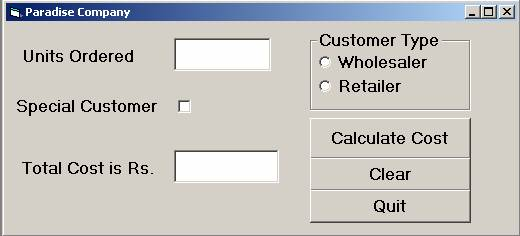Visitors Online: 0 | Monday 09th December 2019CBSE Guess > Papers > Important Questions > Class XII > 2009 > Informatics Practices > Informatics Practices By Mr. Vinay Kumar Srivastava

CBSE CLASS XII

Section – A

Q. 1. Answer the following questions.

1. What is OSS? How is it different from Proprietary software? 2
2. What is SDLC? Name the common fact recording methods. 2
3. What is UML? What does it do? 2
4. How is a back-end linked to a database and a server? 2
5. What for are these software used? 2
6. Mozilla Firefox ii) Linux

Q. 2. Answer the following questions.

1. Name any Four property of MDI from. 2
2. Which loop executes when the given condition is False? Explain with an example. 2
3. What are modules in VB? Name various modules available in VB. 2
4. Define: RecordSet and Record Source. 2
5. What do you mean by scope and life time of variables? Explain with example. 2

Q. 3. (a) State any two advantages of PL/SQL over SQL. 2
b) Differentiate between IN and OUT parameter of PL/SQL procedure. 2
c) State the difference between –
i) DDL and DCL commands. ii) %Type and %Rowtype 2
d) What is trigger? Name the statements for which triggers are executed. 2
e) What is the need of creating cursor in PL/SQL? Name any two attribute of it. 2

Section - B

Q. 4. Read the following case study and answer the questions that follow.

Paradise Company is a dealer of goods. The company’s programmer has made the following from to calculate the total cost of the order of the customers. The total cost is calculated as per the following criteria.

For Units                           Price For wholesalers                   Price for Retailers
1-30                                     Rs. 100/-                                       Rs. 80/-
31-70                                   Rs. 90/-                                         Rs. 70/-
>70                                      Rs. 60/-                                         Rs. 50/-

Special customers are given a discount of 20%.

 Object Type Object Name Description Form FrmParadise Main Form Text Box txtUnitOrdered To enter Unit Ordered txtTotalCost To show calculated cost Check Box chkSpCust To be checked if customer is special Option Button optWholesalerl To Provide customer type optRetailer Command Button cmdCalcCost To calculate total cost cmdClear To clear the entered values cmdQuit To close the application1. Write the commands to disable the text boxes txtPercentage and txtGrade. 1
2. Write the code for text boxes txtFirstTerm or txtSecondTerm which accept only numeric data. 1
3. Write the code for cmdCalcPer to calculate the percentage after finding the total marks of first term and second term (assuming that both marks are out of 100). Also ensure that NCC cadets get an increment of 5% in their percentage. 3
4. Write the code for cmdCalcGrade to calculate the grade depending on the stream selected according to the criteria given above. 3
5. Write the code for cmdClear command button to clear all the text boxes and check box. Also write the code for cmdExit to close the application. 2

Q. 5. (a) Find the error from the following code segment and rewrite the corrected code underlining the correction made(VB):
dim work as integer, I as integer
work=10
I=0
do while work<20 loop
print work * I
I = I + 2
If I = 8 then
Work=20
Elseif
Work=work – 3
end
loop while 2

(b). Find the output of the following statements:

1. Print 9 and 5
2. Print INT(4-8*5/2+10) 2

(c). Rewrite the following Procedure after converting into Function.
Sub Factorial( N as Integer)
Dim Fact as Long
Fact=1
For I = N to 2 Step -1
Fact=Fact*I
Next I
Print “Factorial of”; N; ”is “; Fact
End Sub 2

(d). Write a function which takes a string as argument and return the reverse of it. 2

(e). Write a procedure that takes two arguments and swaps the numbers without using third variable. 2

Section - C

Q. 6. Answer the following question.

(a). Write the output produced by the following part of code in PL/SQL.
DECLARE
Num NUMBER:=3;
BEGIN
FOR I IN 1..3 LOOP
FOR J IN REVERSE 1..2 LOOP
Num:=Num+J;
DBMS_OUTPUT.PUT(Num||’ ‘);
END LOOP;
DBMS_OUTPUT.NEW_LINE;
Num:=Num + MOD(Num,2);
END LOOP;
END; 2

(b). Find Error in the following code, underline errors and rewrite the correct code.
DECLARE;
Bonus NUMBER default 10;
Commission NUMBER default 20;
Status BOOLEAN =True;
BEGIN ;
Bonus<Commission:=Status;
END; 2

(c). What are anonymous blocks? How will you save and execute it. 2

(d). I want to execute a block of code every time when SQL session starts, where I would write the code. 1

(e). Write a PL/SQL function Exponent that takes a 3-digit number argument and return the value, first digit to the power second digit to the power third digit. 3

Q. 7. Answer the following question based on the FLIGHT table.

Table : FLIGHT

 Column Name Data Type Size Constraint Description Flight_No NUMBER 4 Primary Key Flight Number Origin VARCHAR2 30 NOT NULL Place of Origin of Flight Destination VARCHAR2 30 NOT NULL Destination of Flight Seats NUMBER 3 Less than 50 No. of seats Available Flt_Date DATE Sate of Flight Rate NUMBER 7,2 Rate of Ticket
1. Write the SQL command to create the table Flight including the constraints.
2. Write the SQL command to display the details of all the flights whose destination is the same as the destination of Flight_No 3002.
3. Write a PL/SQL procedure IncreaseRate to increase rate by 15% of the flight whose Flight_No is passed in the procedure.
4. Write PL/SQL code to create two statement level triggers BD_FLIGHT and AD_FLIGHT before and after DELETE statement respectively on the table FLIGHT which displays a message ‘Ready for Deletion’ and ‘Record Deleted’ respectively.

Paper By Mr. Vinay Kumar Srivastava